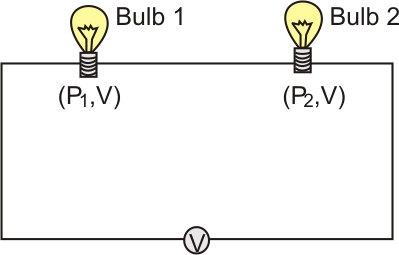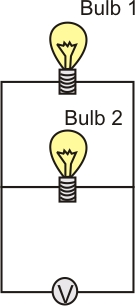#### Two bulbs are relatedand, If they are connected (i) in series    (ii) in parallel across a supplyfind the power dissipated in the two combinations in terms ofand.

Electric power is defined as the rate at which electrical energy is consumed in an electrical circuit. Electric power is given as:-(as)

Where V is the potential differenceis the current andis the Resistance

(i) When two bulbs ratedandare connected in series, the current is the same through each bulb.Ifandbe the resistances of two bulbs, then effective resistance of     the circuit is,Using,we have,We have given that :-Therefore -Thus, the effective power when two bulbs are connected in series is(ii) When two bulbs ratedandare connected in parallel.

using the relation of parallel connection, we haveThus,given :Thus,Therefore, the effective power or power (dissipated) when two bulbs are connected in parallel is#### Safeer PP